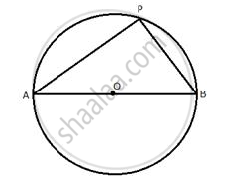# The Locus of a Point P, So That: Ab^2 = Ap^2 + Bp^2 Where a and B Are Two Fixed Points. - Mathematics

Sum

Describe the locus for questions 1 to 13 given below:

The locus of a point P, so that:
AB^2 = AP^2 + BP^2,
Where A and B are two fixed points.

#### SolutionThe locus of the point P is the circumference of a circle with AB as diameter and satisfies the condition AB^2 = AP^2 + BP^2

Concept: Constructions Under Loci
Is there an error in this question or solution?

#### APPEARS IN

Selina Concise Maths Class 10 ICSE
Chapter 16 Loci (Locus and Its Constructions)
Exercise 16 (B) | Q 12 | Page 241